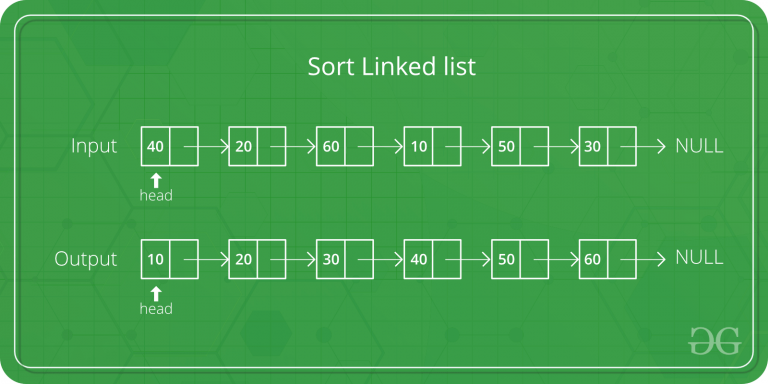# Python Program For QuickSort On Singly Linked List

• Last Updated : 18 May, 2022

QuickSort on Doubly Linked List is discussed here. QuickSort on Singly linked list was given as an exercise. The important things about implementation are, it changes pointers rather swapping data and time complexity is same as the implementation for Doubly Linked List.In partition(), we consider last element as pivot. We traverse through the current list and if a node has value greater than pivot, we move it after tail. If the node has smaller value, we keep it at its current position.

In QuickSortRecur(), we first call partition() which places pivot at correct position and returns pivot. After pivot is placed at correct position, we find tail node of left side (list before pivot) and recur for left list. Finally, we recur for right list.

## Python3

 `# Sort a linked list using quick sort ` `class` `Node: ` `    ``def` `__init__(``self``, val): ` `        ``self``.data ``=` `val ` `        ``self``.``next` `=` `None` ` `  `class` `QuickSortLinkedList: ` `    ``def` `__init__(``self``): ` `        ``self``.head``=``None` ` `  `    ``def` `addNode(``self``, data): ` `        ``if` `(``self``.head ``=``=` `None``): ` `            ``self``.head ``=` `Node(data) ` `            ``return` ` `  `        ``curr ``=` `self``.head ` `        ``while` `(curr.``next` `!``=` `None``): ` `            ``curr ``=` `curr.``next` ` `  `        ``newNode ``=` `Node(data) ` `        ``curr.``next` `=` `newNode ` ` `  `    ``def` `printList(``self``, n): ` `        ``while` `(n !``=` `None``): ` `            ``print``(n.data, end ``=` `" "``) ` `            ``n ``=` `n.``next` ` `  `    ``''' Takes first and last node,but do not ` `        ``break any links in the whole linked list'''` `    ``def` `partitionLast(``self``, start, end): ` `        ``if` `(start ``=``=` `end ``or`  `            ``start ``=``=` `None` `or` `end ``=``=` `None``): ` `            ``return` `start ` ` `  `        ``pivot_prev ``=` `start ` `        ``curr ``=` `start ` `        ``pivot ``=` `end.data ` ` `  `        ``''' Iterate till one before the end,  ` `            ``no need to iterate till the end  ` `            ``because the end is pivot '''` `        ``while` `(start !``=` `end): ` `            ``if` `(start.data < pivot): ` `               `  `                ``# Keep tracks of last  ` `                ``# modified item ` `                ``pivot_prev ``=` `curr ` `                ``temp ``=` `curr.data ` `                ``curr.data ``=` `start.data ` `                ``start.data ``=` `temp ` `                ``curr ``=` `curr.``next` `            ``start ``=` `start.``next` ` `  `        ``''' Swap the position of curr i.e.  ` `            ``next suitable index and pivot'''` `        ``temp ``=` `curr.data ` `        ``curr.data ``=` `pivot ` `        ``end.data ``=` `temp ` ` `  `        ``''' Return one previous to current  ` `            ``because current is now pointing  ` `            ``to pivot '''` `        ``return` `pivot_prev ` ` `  `    ``def` `sort(``self``, start, end): ` `        ``if``(start ``=``=` `None` `or`  `           ``start ``=``=` `end ``or` `start ``=``=` `end.``next``): ` `            ``return` ` `  `        ``# Split list and partition recurse ` `        ``pivot_prev ``=` `self``.partitionLast(start,  ` `                                       ``end) ` `        ``self``.sort(start, pivot_prev) ` ` `  `        ``''' If pivot is picked and moved to  ` `            ``the start, that means start and  ` `            ``pivot is the same so pick from  ` `            ``next of pivot '''` `        ``if``(pivot_prev !``=` `None` `and`  `           ``pivot_prev ``=``=` `start): ` `            ``self``.sort(pivot_prev.``next``, end) ` ` `  `        ``# If pivot is in between of the list,  ` `        ``# start from next of pivot, since we  ` `        ``# have pivot_prev, so we move two nodes ` `        ``elif` `(pivot_prev !``=` `None` `and`  `              ``pivot_prev.``next` `!``=` `None``): ` `            ``self``.sort(pivot_prev.``next``.``next``,  ` `                      ``end) ` ` `  `# Driver code ` `if` `__name__ ``=``=` `"__main__"``: ` `    ``ll ``=` `QuickSortLinkedList() ` `    ``ll.addNode(``30``) ` `    ``ll.addNode(``3``) ` `    ``ll.addNode(``4``) ` `    ``ll.addNode(``20``) ` `    ``ll.addNode(``5``) ` ` `  `    ``n ``=` `ll.head ` `    ``while` `(n.``next` `!``=` `None``): ` `        ``n ``=` `n.``next` ` `  `    ``print``(``"Linked List before sorting"``) ` `    ``ll.printList(ll.head) ` ` `  `    ``ll.sort(ll.head, n) ` ` `  `    ``print``(``"Linked List after sorting"``); ` `    ``ll.printList(ll.head) ` `# This code is contributed by humpheykibet `

Output:

```Linked List before sorting
30 3 4 20 5